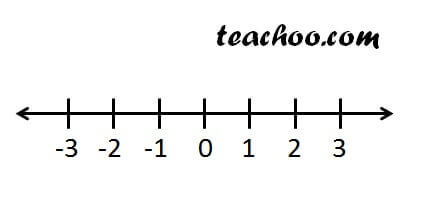Rational numbers - Definition

Chapter 1 Class 9 Number Systems
Concept wise

There are different types of numbers

• Natural Numbers
• Whole Numbers
• Integers
• Rational Numbers
• Irrational Numbers
• Real numbers
• Complex numbers

We can also classify numbers as

• Odd or even
• Prime or composite
• Decimals, or fractions

Let's look at some different type of numbers

## Natural numbers

Numbers which start from 1.

Eg:- 1, 2, 3, 4,………..

## Whole numbers

Numbers which start from 0.

Eg:- 0,1, 2, 3, 4,………..

## Integers

Positive and negative numbers are called integers

Eg:-  ……… -4, -3, -2, -1, 0, 1, 2, 3, 4,………..

## Rational Numbers

Numbers which can be written in p/q form, where q ≠ 0

Eg:- 2/3, 4/5

## Irrational Numbers

Numbers which cannot be expressed in p/q form.

Eg:- √2, √3, π

## Real Numbers

All Numbers on number line are real numbers.It includes rational numbers & irrational numbers both.

There are some numbers which we will study in Class 11,

Learn in your speed, with individual attention - Teachoo Maths 1-on-1 Class

### Transcript

There are different types of numbers Natural Numbers Whole Numbers Integers Rational Numbers Irrational Numbers Real numbers Complex numbers Natural numbers Numbers which start from 1. Eg:- 1, 2, 3, 4,……….. Whole numbers Numbers which start from 0. Eg:- 0,1, 2, 3, 4,……….. Integers Positive and negative numbers are called integers Eg:- ……… -4, -3, -2, -1, 0, 1, 2, 3, 4,……….. Rational Numbers Numbers which can be written in p/q form, where q ≠ 0 Eg:- 2/3, 4/5 Irrational Numbers Numbers which cannot be expressed in p/q form. Eg:- √2, √3, π Real Numbers All Numbers on number line are real numbers. It includes rational numbers & irrational numbers both. There are some numbers which we will study in Class 11, Complex numbers.